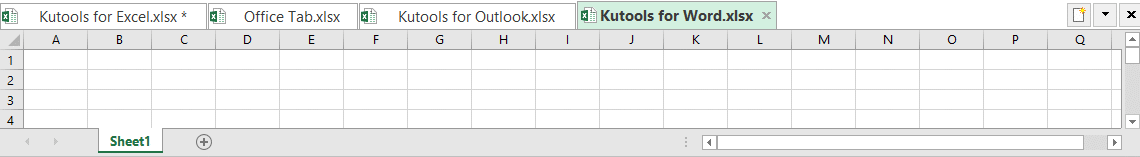## How to count filtered cells with text in Excel?

In Excel, counting cells with text is simple. But when it comes to counting filtered cells with text, things get tricky. This tutorial will introduce three ways to count the cells with text from a filtered list.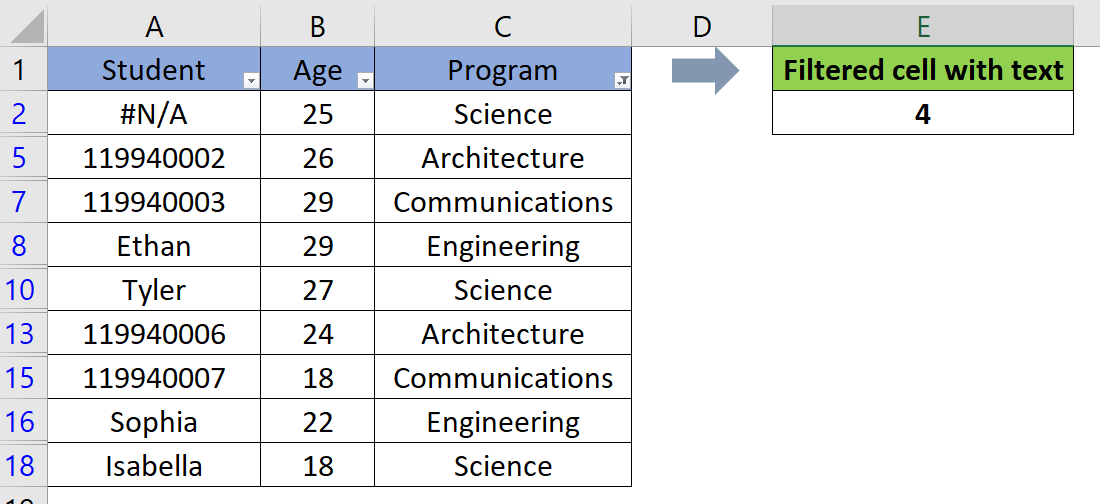Count filtered cells with text by using a helper column

Count filtered cells with text by concatenating SUMPRODUCT, SUBTOTAL, INDIRECT, ROW & ISTEXT Functions

Count filtered cells with text by combining SUMPRODUCT, SUBTOTAL, OFFSET, MIN, ROW & ISTEXT Functions

#### Count filtered cells with text by using a helper column

Using the COUNTIF function, in addition with the help of a helper column, we can easily count the filtered cells with text. Please do as follows.

1. Please copy the formula below into cell D2, then press the Enter key to get the first result.

=SUBTOTAL (103, A2)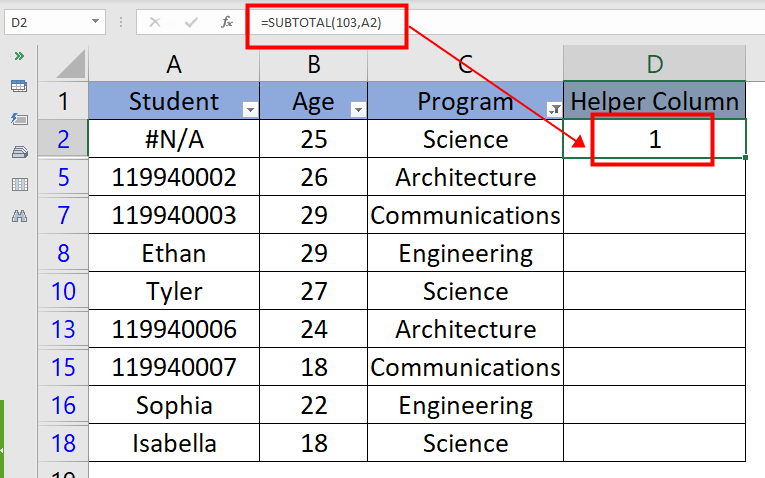Note: The helper column with the SUBTOTAL formula is for testing whether the row is filtered or not. And 103 stands for the COUNTA function in the function_num argument.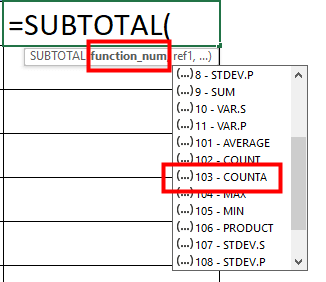2. Then drag the fill handle down to the cells you want to apply this formula too.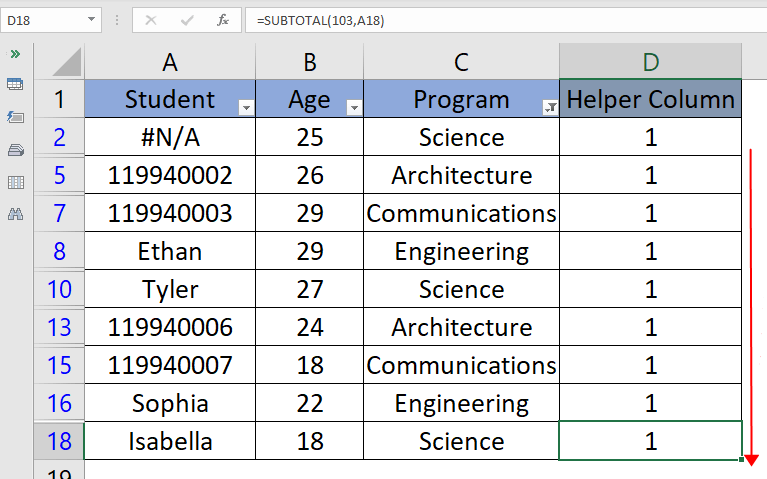3. Please copy the formula below into cell F2, then press the Enter key to get the final result.

=COUNTIFS(A2:A18,"*", D2:D18, 1)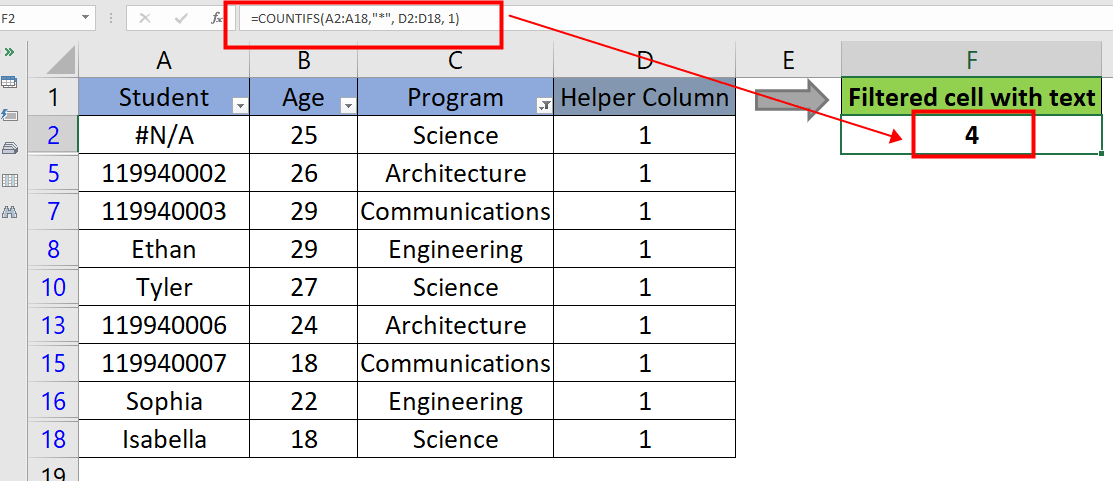We can see that there are 4 cells with text in the filtered data.

#### Count filtered cells with text by concatenating SUMPRODUCT, SUBTOTAL, INDIRECT, ROW & ISTEXT Functions

Another method of counting the filtered cells with text is using the combination of the SUMPRODUCT, SUBTOTAL, INDIRECT, ROW & ISTEXT Functions. Please do as follows.

Please copy the formula below into cell E2, then press the Enter key to get the result.

=SUMPRODUCT(SUBTOTAL(103, INDIRECT("A"&ROW(A2:A18))), --(ISTEXT(A2:A18)))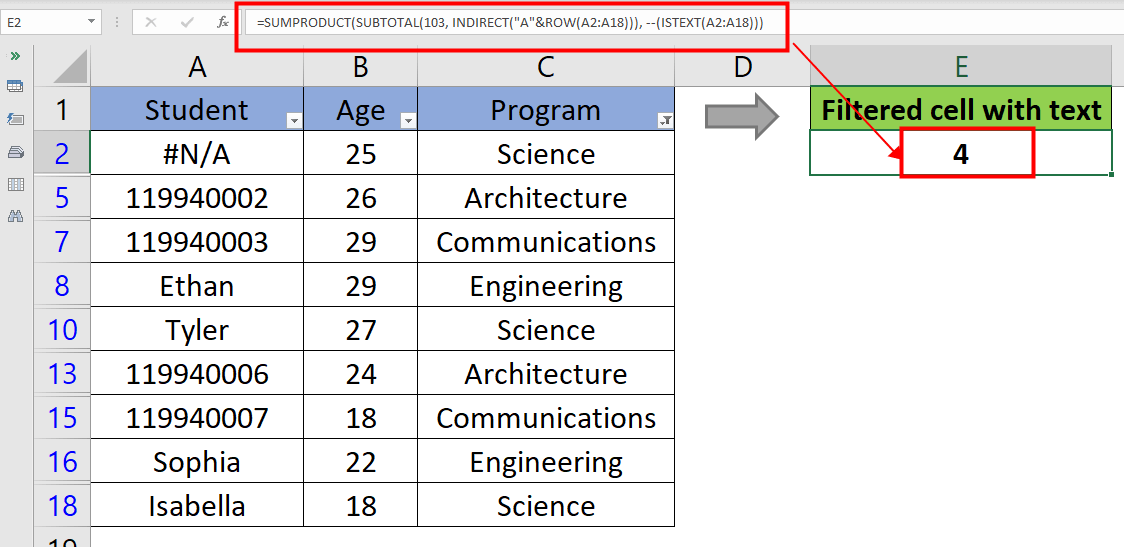##### Formula Explanation:
1. ROW(A2:A18) returns the respective row numbers of the range A2:A18.
2. INDIRECT("A"&ROW(A2:A18)) returns the valid cell references from the given range.
3. SUBTOTAL(103, INDIRECT("A"&ROW(A2:A18))) tests whether the row is filtered or not, and returns 1 for the visible cells, 0 for the hidden and empty cells.
4. ISTEXT(A2:A18) checks if each cell in range A2:A18 contains text, and return True for the cells with text, False for the other cells. The double unary operator (--) coerces the TRUE and FALSE values into 1's and 0's.
5. SUMPRODUCT(SUBTOTAL(103, INDIRECT("A"&ROW(A2:A18))), --(ISTEXT(A2:A18))) can be viewed as SUMPRODUCT({1;1;1;1;1;1;1;1;1}, {0;0;0;1;1;0;0;1;1}). Then the SUMPRODUCT multiplies the two arrays together and returns the sum of values, which is 4.

#### Count filtered cells with text by combining SUMPRODUCT, SUBTOTAL, OFFSET, MIN, ROW & ISTEXT Functions

The third method of counting the cells with text from a filtered data is concatenating the SUMPRODUCT, SUBTOTAL, OFFSET, MIN, ROW & ISTEXT Functions. Please do as follows.

Please copy the formula below into cell E2, then press the Enter key to get the result.

=SUMPRODUCT(SUBTOTAL(103, OFFSET(A2:A18, ROW(A2:A18)-2 -- MIN(ROW(A2:A18)-2),,1)), -- (ISTEXT(A2:A18)))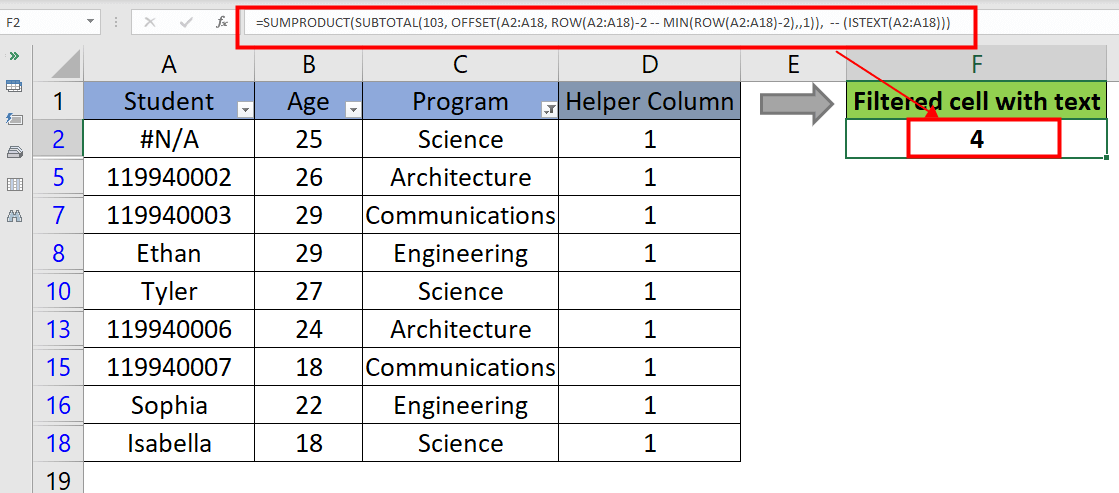##### Formula Explanation:
1. OFFSET(A2:A18, ROW(A2:A18)-2 -- MIN(ROW(A2:A18)-2),,1) returns the individual cell references from range A2:A18.
2. SUBTOTAL(103, OFFSET(A2:A18, ROW(A2:A18)-2 -- MIN(ROW(A2:A18)-2),,1)) checks whether the row is filtered or not, and returns 1 for the visible cells, 0 for the hidden and empty cells.
3. ISTEXT(A2:A18) checks if each cell in range A2:A18 contains text, and return True for the cells with text, False for the other cells. The double unary operator (--) coerces the TRUE and FALSE values into 1's and 0's.
4. SUMPRODUCT(SUBTOTAL(103, OFFSET(A2:A18, ROW(A2:A18)-2 -- MIN(ROW(A2:A18)-2),,1)), -- (ISTEXT(A2:A18))) can be viewed as SUMPRODUCT({1;1;1;1;1;1;1;1;1}, {0;0;0;1;1;0;0;1;1}). Then the SUMPRODUCT multiplies the two arrays together and returns the sum of values, which is 4.

#### Other Operations (Articles)

Combine The COUNTIF And LEFT Functions To Count Cells Begin With A Certain Character In Excel
To count the number of cells which begin with a certain character in Excel, you can combine the COUNTIF and LEFT functions to get it done. Actually there are different kinds of formulas can achieve it. This tutorial will guide you step by step.

How To Count Cells Containing Numbers Or Not In Excel?
If there are a range of cells, some are containing numbers, and the other containing text, how can you count the cells containing numbers or not quickly in Excel?

How To Count Cells If One Of Multiple Criteria Met In Excel?
What if counting cells if contain one of multiple criteria? Here I will share the ways to count cells if contain X or Y or Z … etc. in Excel.

How To Count Cells With Specific Text And Fill/Font Color In Excel?
Do you know how to count cells with multiple conditions? For example, count number of cells that contain both specific text and font/fill color. This article will show you the solution.

## The Best Office Productivity Tools

### Kutools for Excel Solves Most of Your Problems, and Increases Your Productivity by 80%

• Super Formula Bar (easily edit multiple lines of text and formula); Reading Layout (easily read and edit large numbers of cells); Paste to Filtered Range...
• Merge Cells/Rows/Columns and Keeping Data; Split Cells Content; Combine Duplicate Rows and Sum/Average... Prevent Duplicate Cells; Compare Ranges...
• Select Duplicate or Unique Rows; Select Blank Rows (all cells are empty); Super Find and Fuzzy Find in Many Workbooks; Random Select...
• Exact Copy Multiple Cells without changing formula reference; Auto Create References to Multiple Sheets; Insert Bullets, Check Boxes and more...
• Favorite and Quickly Insert Formulas, Ranges, Charts and Pictures; Encrypt Cells with password; Create Mailing List and send emails...
• Extract Text, Add Text, Remove by Position, Remove Space; Create and Print Paging Subtotals; Convert Between Cells Content and Comments...
• Super Filter (save and apply filter schemes to other sheets); Advanced Sort by month/week/day, frequency and more; Special Filter by bold, italic...
• Combine Workbooks and WorkSheets; Merge Tables based on key columns; Split Data into Multiple Sheets; Batch Convert xls, xlsx and PDF...
• Pivot Table Grouping by week number, day of week and more... Show Unlocked, Locked Cells by different colors; Highlight Cells That Have Formula/Name...### Office Tab - brings tabbed interface to Office, and make your work much easier

• Enable tabbed editing and reading in Word, Excel, PowerPoint, Publisher, Access, Visio and Project.
• Open and create multiple documents in new tabs of the same window, rather than in new windows.
• Increases your productivity by 50%, and reduces hundreds of mouse clicks for you every day!